## Saturday, January 31, 2009

### Magnetic Circuits

What is a magnetic circuit? To better understand magnetic circuits, a basic understanding of the physical qualities of magnetic circuits will be necessary.

A magnetic circuit can be compared with an electric current in which EMF, or voltage, produces a current flow. The ampere-turns (NI), or the magnetomotive force (Fm or mmf), will produce a magnetic flux phi(Figure 1). The mmf can be compared with EMF, and the flux (phi) can be compared to current. Equation (1) is the mathematical representation of magnetomotive force derived using Ohm’s Law, I = E / R.

phi = Fm / R = mmf / R……….(1)

where
phi = magnetic flux, Wb
Fm = magnetomotive force (mmf), At
R = reluctance, At/Wb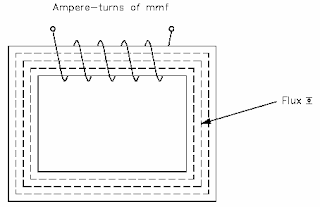Figure 1 Magnetic Current with Closed Iron Path

Equation (2) is the mathematical representation for reluctance.

R = L / µA…………………….(2)

where
R = reluctance, At/Wb
L = length of coil, m
µ = permeability of magnetic material, (T-m)/At
A = cross-sectional area of coil, m^2

Example: A coil has an mmf of 600 At, and a reluctance of 3 x 10^6 At/Wb.
Find the total flux phi.
Solution:
flux phi = mmf/R = 600At / (3 x 10^6 At/Wb) = 200 x 10^-6 Wb = 200 µWb

BH Magnetization Curve

The BH Magnetization Curve (Figure 2) shows how much flux density (B) results from increasing the flux intensity (H). The curves in Figure 2 are for two types of soft iron cores plotted for typical values. The curve for soft iron 1 shows that flux density B increases rapidly with an increase in flux intensity H, before the core saturates, or develops a "knee." Thereafter, an increase in flux intensity H has little or no effect on flux density B. Soft iron 2 needs a much larger increase in flux intensity H before it reaches its saturation level at H = 5000 At/m, B = 0.3 T.

Air, which is nonmagnetic, has a very low BH profile, as shown in Figure 2.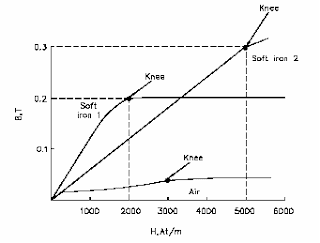Figure 2 Typical BH Curve for Two Types of Soft Iron

The permeability (µ) of a magnetic material is the ratio of B to H. Equation (3) is the mathematical representation for magnetic material permeability.

µ = B / H………(3)

The average value of permeability is measured where the saturation point, or knee, is first established. Figure 27 shows that the normal or average permeability for the two irons as follows.

µ soft iron 1 = B / H = 0.2 / 2000 = 1 x 10^-4 (T m)/At
µ soft iron 2 = B / H = 0.3 / 5000 = 6 x 10^-5 (T m)/At

In SI units, the permeability of a vacuum is µo = 4 x 3.14 x 10^-7 H/m or 1.26 x 10^-6 or T-m/At (3.14 is phi). In order to calculate permeability, the value of relative permeability µr must be multiplied by µo. Equation (4) is the mathematical representation for permeability.

µ = µr x µo…………….(4)

Example: Find the permeability of a material that has a relative permeability of 100.
µ = µr x µo = 100 (1.26 x 10^-6) = 126 x 10^-6 (T m)/At

Hysteresis

When current in a coil reverses direction thousands of times per second, hysteresis can cause considerable loss of energy. Hysteresis is defined as "a lagging behind." The magnetic flux in an iron core lags behind the magnetizing force.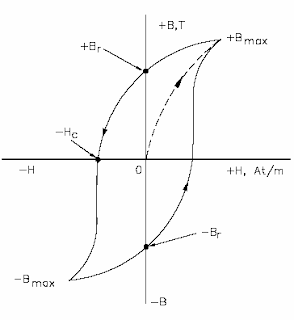Figure 3 Hysteresis Loop for Magnetic Materials

The hysteresis loop is a series of curves that shows the characteristics of a magnetic material (Figure 3). Opposite directions of current will result in opposite directions of flux intensity shown as +H and -H. Opposite polarities are also shown for flux density as +B or -B. Current starts at the center (zero) when unmagnetized. Positive H values increase B to the saturation point, or +Bmax, as shown by the dashed line. Then H decreases to zero, but B drops to the value of Br due to hysteresis. By reversing the original current, H now becomes negative. B drops to zero and continues on to -Bmax. As the -H values decrease (less negative), B is reduced to –Br when H is zero. With a positive swing of current, H once again becomes positive, producing saturation at +Bmax. The hysteresis loop is completed. The loop does not return to zero because of hysteresis. The value of +Br or -Br, which is the flux density remaining after the magnetizing force is zero, is called the retentivity of that magnetic material. The value of -Hc, which is the force that must be applied in the reverse direction to reduce flux density to zero, is called the coercive force of the material. The greater the area inside the hysteresis loop, the larger the hysteresis losses.

Magnetic Induction

Electromagnetic induction was discovered by Michael Faraday in 1831. Faraday found that if a conductor "cuts across" lines of magnetic force, or if magnetic lines of force cut across a conductor, a voltage, or EMF, is induced into the conductor. Consider a magnet with its lines of force from the North Pole to the South Pole (Figure 4). A conductor C, which can be moved between the poles of the magnet, is connected to a galvanometer G, which can detect the presence of voltage, or EMF. When the conductor is not moving, zero EMF is indicated by the galvanometer.

If the conductor is moving outside the magnetic field at position 1, zero EMF is still indicated by the galvanometer. When the conductor is moved to position 2, the lines of magnetic force will be cut by the conductor, and the galvanometer will deflect to point A. Moving the conductor to position 3 will cause the galvanometer to return to zero. By reversing the direction in which the conductor is moved (3 to 1), the same results are noticed, but of opposite polarity. If we hold the conductor stationary in the magnetic lines of force, at position 2, the galvanometer indicates zero. This fact shows that there must be relative motion between the conductor and the magnetic lines of force in order to induce an EMF.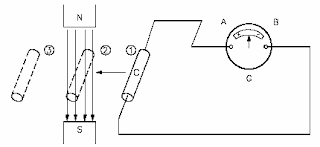Figure 4 Induced EMF

The most important application of relative motion is seen in electric generators. In a DC generator, electromagnets are arranged in a cylindrical housing. Conductors, in the form of coils, are rotated on a core such that the coils continually cut the magnetic lines of force. The result is a voltage induced in each of the conductors. These conductors are connected in series, and the induced voltages are added together to produce the generator’s output voltage.

The magnitude of the induced voltage depends on two factors: (1) the number of turns of a coil, and (2) how fast the conductor cuts across the magnetic lines of force, or flux. Equation (5) is the mathematical representation for Faraday’s Law of Induced Voltage.

Vind = N (delta.phi/delta.t).......(5)

Where

Vind = induced voltage, V

N = number of turns in a coil
delta.phi/delta.t= rate at which the flux cuts across the conductor, Wb/s

Example 1: Given: Flux = 4 Wb. The flux increases uniformly to 8 Wb in a period of 2 seconds. Find induced voltage in a coil that has 12 turns, if the coil is stationary in the magnetic field.

Solution:

Vind = N(d.phi/d.t)
delta.phi = 8Wb - 4Wb = 4Wb
d.t = 2s

then
delta.phi/delta.t = 4Wb/2s = 2Wb/s
Vind = -12 (2) = -24 volts

Example 2: In Example 1, what is the induced voltage, if the flux remains 4 Wb after 2s ?

Solution:
Vind = -12(0/2) = 0 Volts

*) No voltage is induced in Example 2. This confirms the principle that relative motion must exist between the conductor and the flux in order to induce a voltage.

Lenz’s Law

Lenz’s Law determines the polarity of the induced voltage. Induced voltage has a polarity that will oppose the change causing the induction. When current flows due to the induced voltage, a magnetic field is set up around that conductor so that the conductor’s magnetic field reacts with the external magnetic field. This produces the induced voltage to oppose the change in the external magnetic field. The negative sign in equation (5) is an indication that the emf is in such a direction as to produce a current whose flux, if added to the original flux, would reduce the magnitude of the emf.

Summary

The important information contained in this chapter is summarized below.
Simple magnetic circuit magnetic flux (phi) is proportional to the magnetomotive force (Fm) and indirectly proportional to the reluctance (R) in a circuit.

phi(Wb) = Fm (At) / R (At/Wb)

A BH magnetization curve shows how much magnetic flux density (B) results from increasing magnetic flux intensity. The "knee" identifies the point where increasing flux intensity (H) results in a minimal increase in flux density (B). Hysteresis losses are caused by reversing current direction thousands of times in a coil.

Faraday’s Law of Induced Voltage depends on the number of turns of a coil and how fast the conductor cuts across the magnetic lines of force or flux.
Vind = -N(delta.phi/delta.t)

Lenz’s Law states that an induced voltage will have a polarity that will oppose the magnetic field that caused the induced voltage.

### Magnetism

Certain metals and metallic oxides have the ability to attract other metals. This property is called magnetism, and the materials which have this property are called magnets. Some magnets are found naturally while others must be manufactured.

Magnetism is a result of electrons spinning on their own axis around the nucleus (Figure 1).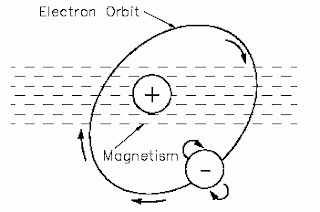Figure 1 Electron Spinning Around Nucleus Produces Magnetic Field

In magnetic materials, the atoms have certain areas called domains. These domains are aligned such that their electrons tend to spin in the same direction (Figure 2).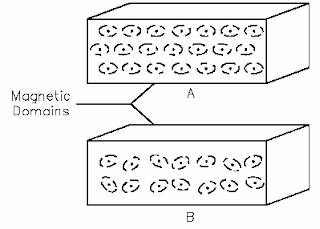Figure 2 Magnetic Domains

The alignment of these domains results in the formation of magnetic poles at each end of the magnet. These poles are called the north pole and the south pole. The law of magnetism states that like magnetic poles repel and unlike magnetic poles attract one another (Figure 3).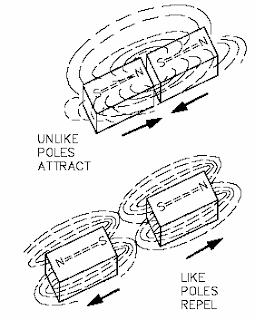Figure 3 The Law of Magnetic Attraction and Repulsion

Magnetic Flux

The group of magnetic field lines emitted outward from the north pole of a magnet is called magnetic flux. The symbol for magnetic flux is (phi). The SI unit of magnetic flux is the weber (Wb). One weber is equal to 1 x 108 magnetic field lines.

Example: If a magnetic flux (phi) has 5,000 lines, find the number of webers.

Phi = (5000 lines)/(1 x 108 lines/Wb) = (5 x 10^3)/10^8 = 50 x 10^-6 Wb = 50 µWb

Magnetic Flux Density

Magnetic flux density is the amount of magnetic flux per unit area of a section, perpendicular to the direction of flux. Equation (1) is the mathematical representation of magnetic flux density.

B = phi/A……..(1)

where
B = magnetic flux density in teslas (T)
phi= magnetic flux in webers (Wb)
A = area in square meters (m^2)

The result is that the SI unit for flux density is webers per square meter (Wb/m^2). One weber per square meter equals one tesla.

Example: Find the flux density in teslas, when the flux is 800 µWb and the area is 0.004 m^2
Given: phi = 800 µWb = 8 x 10^-4Wb
A = 0.0004 m^2 = 4 x 10^-4m^2
B = phi / A = 8 x 10^-4 Wb/4 x 10^-4m^2 = 2 Wb/m^2

Magnetic Materials

Magnetic materials are those materials that can be either attracted or repelled by a magnet and can be magnetized themselves. The most commonly used magnetic materials are iron and steel. A permanent magnet is made of a very hard magnetic material, such as cobalt steel, that retains its magnetism for long periods of time when the magnetizing field is removed. A temporary magnet is a material that will not retain its magnetism when the field is removed.

Permeability (µ) refers to the ability of a material to concentrate magnetic lines of flux. Those materials that can be easily magnetized are considered to have a high permeability. Relative permeability is the ratio of the permeability of a material to the permeability of a vacuum (µo).
The symbol for relative permeability is µR (mu).

µR = µo/ µo , where µo = 4 x 3.14 x 10^-7H/m …….(2)

Magnetic materials are classified as either magnetic or nonmagnetic based on the highly magnetic properties of iron. Because even weak magnetic materials may serve a useful purpose in some applications, classification includes the three groups described below.

Ferromagnetic Materials: Some of the ferromagnetic materials used are iron, steel, nickel, cobalt, and the commercial alloys, alnico and peralloy. Ferrites are nonmagnetic, but have the ferromagnetic properties of iron. Ferrites are made of ceramic material and have relative permeabilities that range from 50 to 200. They are commonly used in the coils for RF (radio frequency) transformers.

Paramagnetic Materials: These are materials such as aluminum, platinum, manganese, and chromium. These materials have a relative permeability of slightly more than one.
Diamagnetic Materials: These are materials such as bismuth, antimony, copper, zinc, mercury, gold, and silver. These materials have a relative permeability of less than one.

Diamagnetic Materials: These are materials such as bismuth, antimony, copper, zinc, mercury, gold, and silver. These materials have a relative permeability of less than one.

Electromagnetism

The relationship between magnetism and electrical current was discovered by a Danish scientist named Oersted in 1819. He found that if an electric current was caused to flow through a conductor, the conductor produced a magnetic field around that conductor (Figure 4).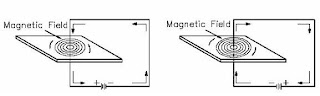Figure 4 The Magnetic Field Produced by Current in a Conductor

Polarity of a Single Conductor

A convenient way to determine the relationship between the current flow through a conductor and the direction of the magnetic lines of force around the conductor is the left-hand rule for current carrying conductors, as illustrated in Figure 5. The student should verify that the lefthand rule holds true for the examples shown in Figure 4.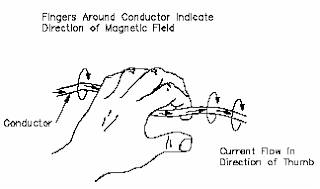Figure 5 Left-hand Rule for Current Carrying Conductors

Magnetic Field and Polarity of a Coil

Bending a straight conductor into a loop has two results: (1) magnetic field lines become more dense inside the loop, and (2) all lines inside the loop are aiding in the same direction. When a conductor is shaped into several loops, it is considered to be a coil. To determine the polarity of a coil, use the left-hand rule for coils (Figure 6).

Adding an iron core inside of a coil will increase the flux density. The polarity of the iron core will be the same as that of the coil. Current flow is from the negative side of the voltage source, through the coil, and back to the positive side of the source (Figure 7).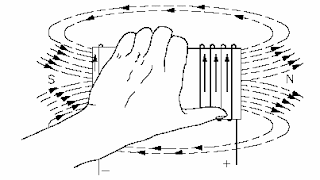Figure 6 Left-hand Rule to Find North Pole of an Electromagnet

Magnetomotive Force

Magnetomotive force (mmf) is the strength of a magnetic field in a coil of wire. This is dependent on how much current flows in the turns of coil: the more current, the stronger the magnetic field; the more turns of wire, the more concentrated the lines of force. The current times the number of turns of the coil is expressed in units called "ampere-turns" (At), also known as mmf. Equation (3) is the mathematical representation for ampere-turns (At).
Fm = ampere-turns = NI………(3)

where
Fm = magnetomotive force (mmf)
N = number of turns
I = current

Example: Calculate the ampere-turns for a coil with 1000 turns and a 5 mA current.
N = 1000 turns and I = 5 mA
substitute
N = 1000 turns and I = 5 x 10^-3
NI = 1000 (5 x 10^-3) = 5 At

Field Intensity

When a coil with a certain number of ampere-turns is stretched to twice its length, the magnetic field intensity, or the concentration of its magnetic lines of force, will be half as great. Therefore, field intensity depends on the length of the coil. Equation (1-14) is the mathematical representation for field intensity, which is related to magnetomotive force as shown.

H = FM / L = NI / L………………..(4)
where
H = field intensity, At/m
NI = ampere-turns (At)
L = length between poles of coil (m)
FM = Magnetomotive force (mmf)

Example 1: Find field intensity of an 80 turn, 20 cm coil, with 6A of current.
Solution:
N = 80, I = 6A, and NI = 480 At
H = 480 At / 0.2 m = 2400 At/m

Example 2: If the same coil in Example 1 were to be stretched to 40 cm with wire length and current remaining the same, find the new value of field intensity.
Solution:
N = 80, I = 6A, and NI = 480 At
H = 480 At / 0.4 m = 1200 At/m
Example 3: The 20 cm coil used in Example 1 with the same current is now wound around an iron core 40 cm in length. Find the field intensity.
Solution:
N = 80, I = 6A, and NI = 480 At
H = 480 At / 0.4 m = 1200 At/m

*) Note that field intensity for Examples 2 and 3 is the same.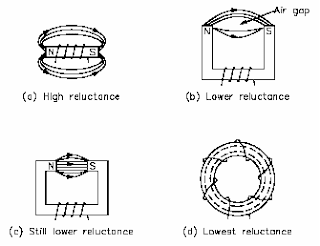Figure 7 Different Physical Forms of Electromagnets

Reluctance

Opposition to the production of flux in a material is called reluctance, which corresponds to resistance. The symbol for reluctance is R, and it has the units of ampere-turns per weber (At/wb).
Reluctance is related to magnetomotive force, mmf, and flux, phi, by the relationship shown in equation (5).

R = mmf / phi ........(5)

Reluctance is inversely proportional to permeability (µ). Iron cores have high permeability and, therefore, low reluctance. Air has a low permeability and, therefore, a high reluctance. Generally, different types of materials have different values of reluctance (Figure 25). Air gap is the air space between two poles of a magnet. Since air has a very high reluctance, the size of the air gap affects the value of reluctance: the shorter the air gap, the stronger the field in the gap. Air is nonmagnetic and will not concentrate magnetic lines. The larger air gap only provides space for the magnetic lines to spread out.

Summary

The important information contained in this chapter is summarized below.
• Magnetic flux - group of magnetic field lines that are emitted outward from the north pole of a magnet
• Magnetic flux density - amount of magnetic flux per unit area of a section, perpendicular to the direction of the flux
• Weber - measure of magnetic flux
• Permeability - ability of a material to concentrate magnetic lines of flux
• Ferromagnetic materials - iron, steel, nickel, cobalt, and commercial alloys with relative permeability ranging from 50-200
• Paramagnetic materials - aluminum, platinum, manganese, and chromium with relative permeability of slightly more than one
• Diamagnetic materials - bismuth, antimony, copper, zinc, mercury, gold, and silver with relative permeability of less than one
• Magnetomotive force (mmf) - strength of a magnetic field in a coil of wire dependent on current flowing through coil
• Ampere turns - current flowing through a coil times the number of turns in the coil
• Field intensity - identifies the magnetic flux density per unit length of a coil
• Reluctance - opposition to the production of flux in a material

### Methods Of Producing Voltage (Electricity)

• Electrochemistry
• Static (friction)
• Induction (magnetism)
• Piezoelectric (pressure)
• Thermal (heat)
• Light
• Thermionic emission

Electrochemistry

Chemicals can be combined with certain metals to cause a chemical reaction that will transfer electrons to produce electrical energy. This process works on the electrochemistry principle. One example of this principle is the voltaic chemical cell, shown in Figure 1. A chemical reaction produces and maintains opposite charges on two dissimilar metals that serve as the positive and negative terminals. The metals are in contact with an electrolyte solution. Connecting together more than one of these cells will produce a battery.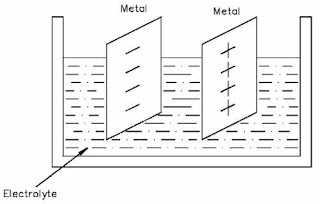Figure 1 Voltaic Chemical Cell

Example: A battery can maintain a potential difference between its positive and negative terminals by chemical action. Various types of cells and batteries will be studied in more detail in Module Batteries.

Static Electricity

Atoms with the proper number of electrons in orbit around them are in a neutral state, or have a "zero charge." A body of matter consisting of these atoms will neither attract nor repel other matter that is in its vicinity. If electrons are removed from the atoms in this body of matter, as happens due to friction when one rubs a glass rod with a silk cloth, it will become electrically positive as shown in Figure 2. If this body of matter (e.g., glass rod) comes near, but not in contact with, another body having a normal charge, an electric force is exerted between them because of their unequal charges. The existence of this force is referred to as static electricity or electrostatic force.
<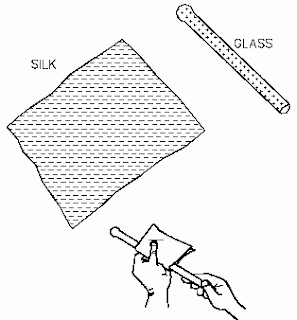Figure 2 Static Electricity

Example: Have you ever walked across a carpet and received a shock when you touched a metal door knob? Your shoe soles built up a charge by rubbing on the carpet, and this charge was transferred to your body. Your body became positively charged and, when you touched the zero-charged door knob, electrons were transferred to your body until both you and the door knob had equal charges.

Magnetic Induction

A generator is a machine that converts mechanical energy into electrical energy by using the principle of magnetic induction. Magnetic induction is used to produce a voltage by rotating coils of wire through a stationary magnetic field, as shown in Figure 3, or by rotating a magnetic field through stationary coils of wire. This is one of the most useful and widelyemployed applications of producing vast quantities of electric power.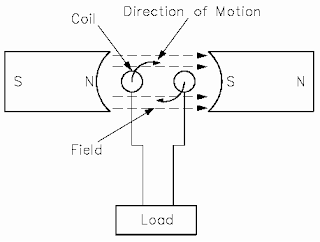Figure 3 Generator – Electromagnetics Induction

Magnetic induction will be studied in more detail in the next two chapters "Magnetism," and "Magnetic Circuits."

Piezoelectric Effect

By applying pressure to certain crystals (such as quartz or Rochelle salts) or certain ceramics (like barium titanate), electrons can be driven out of orbit in the direction of the force. Electrons leave one side of the material and accumulate on the other side, building up positive and negative charges on opposite sides, as shown in Figure 4. When the pressure is released, the electrons return to their orbits. Some materials will react to bending pressure, while others will respond to twisting pressure. This generation of voltage is known as the piezoelectric effect. If external wires are connected while pressure and voltage are present, electrons will flow and current will be produced. If the pressure is held constant, the current will flow until the potential difference is equalized.

When the force is removed, the material is decompressed and immediately causes an electric force in the opposite direction. The power capacity of these materials is extremely small. However, these materials are very useful because of their extreme sensitivity to changes of mechanical force.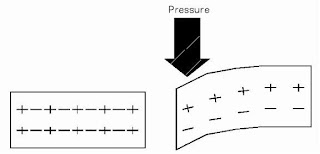Figure 4 Pressure Applied to Certain Crystals Produces an Electric Charge

Example: One example is the crystal phonograph cartridge that contains a Rochelle salt crystal. A phonograph needle is attached to the crystal. As the needle moves in the grooves of a record, it swings from side to side, applying compression and decompression to the crystal. This mechanical motion applied to the crystal generates a voltage signal that is used to reproduce sound.
Thermoelectricity

Some materials readily give up their electrons and others readily accept electrons. For example, when two dissimilar metals like copper and zinc are joined together, a transfer of electrons can take place. Electrons will leave the copper atoms and enter the zinc atoms. The zinc gets a surplus of electrons and becomes negatively charged. The copper loses electrons and takes on a positive charge. This creates a voltage potential across the junction of the two metals. The heat energy of normal room temperature is enough to make them release and gain electrons, causing a measurable voltage potential. As more heat energy is applied to the junction, more electrons are released, and the voltage potential becomes greater, as shown in Figure 5. When heat is removed and the junction cools, the charges will dissipate and the voltage potential will decrease. This process is called thermoelectricity. A device like this is generally referred to as a "thermocouple."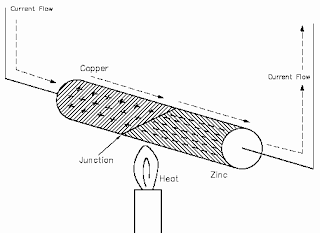Figure 5 Heat Energy Causes Copper to Give up Electrons to Zinc

The thermoelectric voltage in a thermocouple is dependent upon the heat energy applied to the junction of the two dissimilar metals. Thermocouples are widely used to measure temperature and as heat-sensing devices in automatic temperature controlled equipment.
Thermocouple power capacities are very small compared to some other sources, but are
somewhat greater than those of crystals. Generally speaking, a thermocouple can be subjected to higher temperatures than ordinary mercury or alcohol thermometers.

Photoelectric Effect

Light is a form of energy and is considered by many scientists to consist of small particles of energy called photons. When the photons in a light beam strike the surface of a material, they release their energy and transfer it to the atomic electrons of the material. This energy transfer may dislodge electrons from their orbits around the surface of the substance. Upon losing electrons, the photosensitive (light sensitive) material becomes positively charged and an electric force is created, as shown in Figure 6.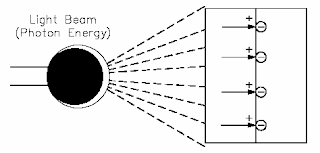Figure 6 Producing Electricity from Light Using a Photovoltaic Cell

This phenomenon is called the photoelectric effect and has wide applications in electronics, such as photoelectric cells, photovoltaic cells, optical couplers, and television camera tubes. Three uses of the photoelectric effect are described below. Photovoltaic: The light energy in one of two plates that are joined together causes one plate to release electrons to the other. The plates build up opposite charges, like a battery (Figure 6). Photoemission: The photon energy from a beam of light could cause a surface to release electrons in a vacuum tube. A plate would then collect the electrons. Photoconduction: The light energy applied to some materials that are normally poor conductors causes free electrons to be produced in the materials so that they become better conductors.

Thermionic Emission

A thermionic energy converter is a device consisting of two electrodes placed near one another in a vacuum. One electrode is normally called the cathode, or emitter, and the other is called the anode, or plate. Ordinarily, electrons in the cathode are prevented from escaping from the surface by a potential-energy barrier. When an electron starts to move away from the surface, it induces a corresponding positive charge in the material, which tends to pull it back into the surface. To escape, the electron must somehow acquire enough energy to overcome this energy barrier. At ordinary temperatures, almost none of the electrons can acquire enough energy to escape. However, when the cathode is very hot, the electron energies are greatly increased by thermal motion. At sufficiently high temperatures, a considerable number of electrons are able to escape. The liberation of electrons from a hot surface is called thermionic emission.

The electrons that have escaped from the hot cathode form a cloud of negative charges near it called a space charge. If the plate is maintained positive with respect to the cathode by a battery, the electrons in the cloud are attracted to it. As long as the potential difference between the electrodes is maintained, there will be a steady current flow from the cathode to the plate. The simplest example of a thermionic device is a vacuum tube diode in which the only electrodes are the cathode and plate, or anode, as shown in Figure 7. The diode can be used to convert alternating current (AC) flow to a pulsating direct current (DC) flow.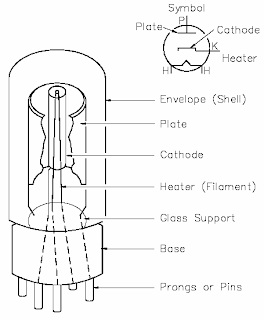Figure 7 Vacuum Tube Diode

Summary